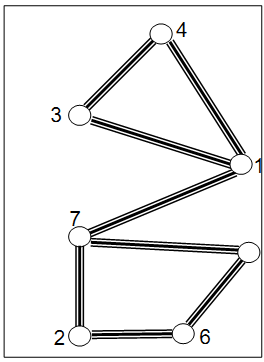# POJ 3895 Cycles of Lanes(DFS)

Cycles of Lanes
 Time Limit: 1000MS Memory Limit: 65536K Total Submissions: 1062 Accepted: 392

Description

Each of the M lanes of the Park of Polytechnic University of Bucharest connects two of the N crossroads of the park (labeled from 1 to N). There is no pair of crossroads connected by more than one lane and it is possible to pass from each crossroad to each other crossroad by a path composed of one or more lanes. A cycle of lanes is simple when passes through each of its crossroads exactly once.
The administration of the University would like to put on the lanes pictures of the winners of Regional Collegiate Programming Contest in such way that the pictures of winners from the same university to be on the lanes of a same simple cycle. That is why the administration would like to assign the longest simple cycles of lanes to most successful universities. The problem is to find the longest cycles? Fortunately, it happens that each lane of the park is participating in no more than one simple cycle (see the Figure).Input

On the first line of the input file the number T of the test cases will be given. Each test case starts with a line with the positive integers N and M, separated by interval (4 <= N <= 4444). Each of the next M lines of the test case contains the labels of one of the pairs of crossroads connected by a lane.

Output

For each of the test cases, on a single line of the output, print the length of a maximal simple cycle.

Sample Input

1
7 8
3 4
1 4
1 3
7 1
2 7
7 5
5 6
6 2

Sample Output

4

Source

#include<cstdio>
#include<cstring>
#include<vector>
#include<algorithm>
using namespace std;
const int N = 4500;
vector<int> ma[N];
int vis[N], a, b, cas, n, m, ans;
int dfs (int i, int no)
{
vis[i] = no;
for (int j = 0; j < ma[i].size(); ++j)
{
if (!vis[ma[i][j]]) dfs (ma[i][j], no + 1);
else ans = max (ans, no - vis[ma[i][j]] + 1);
}
}

int main()
{
scanf ("%d", &cas);
while (cas--)
{
scanf ("%d%d", &n, &m);
for (int i = 1; i <= n; ++i) ma[i].clear();
for (int i = 1; i <= m; ++i)
{
scanf ("%d%d", &a, &b);
ma[a].push_back (b);
ma[b].push_back (a);
}
ans = 0;
memset (vis, 0, sizeof (vis));
for (int i = 1; i <= n; ++i)
if (!vis[i]) dfs (i, 1);
if (ans >= 3) printf ("%d\n", ans);
else printf ("0\n");
}
return 0;
}